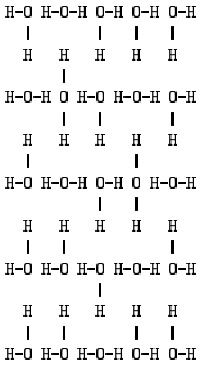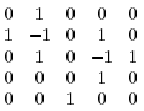Online JudgeProblem SetAuthorsOnline ContestsUser
Web Board
F.A.Qs
Statistical Charts
Problems
Submit Problem
Online Status
Prob.ID:
Register
Authors ranklist
Current Contest
Past Contests
Scheduled Contests
Award Contest
Register
Language:
Square Ice
 Time Limit: 1000MS Memory Limit: 10000K Total Submissions: 4889 Accepted: 1884

Description

Square Ice is a two-dimensional arrangement of water molecules H2O, with oxygen at the vertices of a square lattice and one hydrogen atom between each pair of adjacent oxygen atoms. The hydrogen atoms must stick out on the left and right sides but are not allowed to stick out the top or bottom. One 5 x 5 example is shown below.Note that each hydrogen atom is attached to exactly one of its neighboring oxygen atoms and each oxygen atom is attached to two of its neighboring hydrogen atoms. (Recall that one water molecule is a unit of one O linked to two H's.)

It turns out we can encode a square ice pattern with what is known as an alternating sign matrix (ASM): horizontal molecules are encoded as 1, vertical molecules are encoded as -1 and all other molecules are encoded as 0. So, the above pattern would be encoded as:An ASM is a square matrix with entries 0, 1 and -1, where the sum of each row and column is 1 and the non-zero entries in each row and in each column must alternate in sign. (It turns out there is a one-to-one correspondence between ASM's and square ice patterns!)

Your job is to display the square ice pattern, in the same format as the example above, for a given ASM. Use dashes (-) for horizontal attachments and vertical bars (|) for vertical attachments. The pattern should be surrounded with a border of asterisks (*), be left justified and there should be exactly one character between neighboring hydrogen atoms (H) and oxygen atoms (O): either a space, a dash or a vertical bar.

Input

Input consists of multiple cases. Each case consists of a positive integer m (<= 11) on a line followed by m lines giving the entries of an ASM. Each line gives a row of the ASM with entries separated by a single space. The end of input is indicated by a line containing m = 0.

Output

For each case, print the case number (starting from 1), in the format shown in the Sample Output, followed by a blank line, followed by the corresponding square ice pattern in the format described above. Separate the output of different cases by a blank line.

Sample Input

```2
0 1
1 0
4
0 1 0 0
1 -1 0 1
0 0 1 0
0 1 0 0
0```

Sample Output

```Case 1:

***********
*H-O H-O-H*
*  |      *
*  H   H  *
*      |  *
*H-O-H O-H*
***********

Case 2:

*******************
*H-O H-O-H O-H O-H*
*  |       |   |  *
*  H   H   H   H  *
*      |          *
*H-O-H O H-O H-O-H*
*      |   |      *
*  H   H   H   H  *
*  |           |  *
*H-O H-O H-O-H O-H*
*      |          *
*  H   H   H   H  *
*  |       |   |  *
*H-O H-O-H O-H O-H*
*******************```

Source

[Submit]   [Go Back]   [Status]   [Discuss]Home PageGo BackTo top